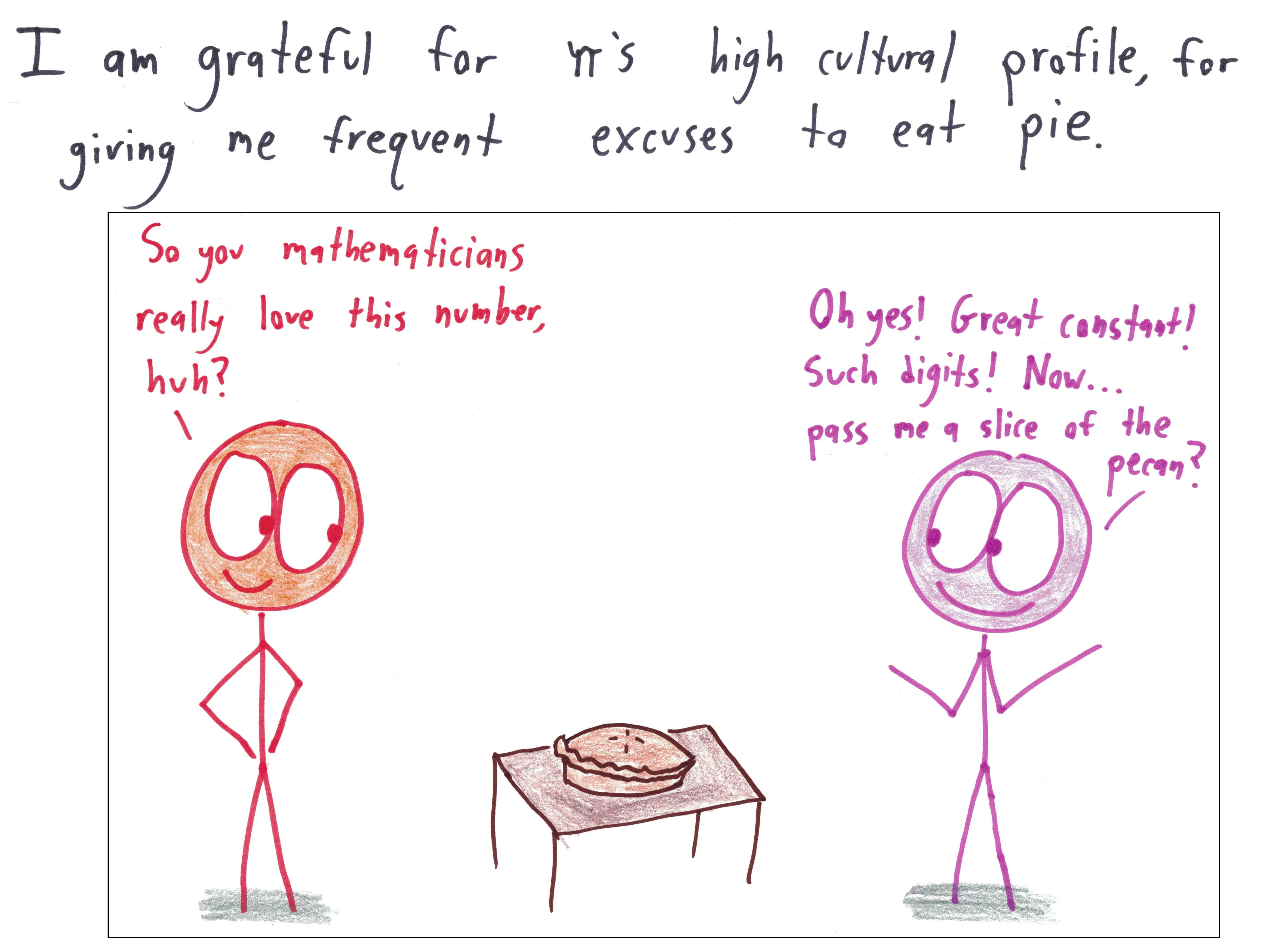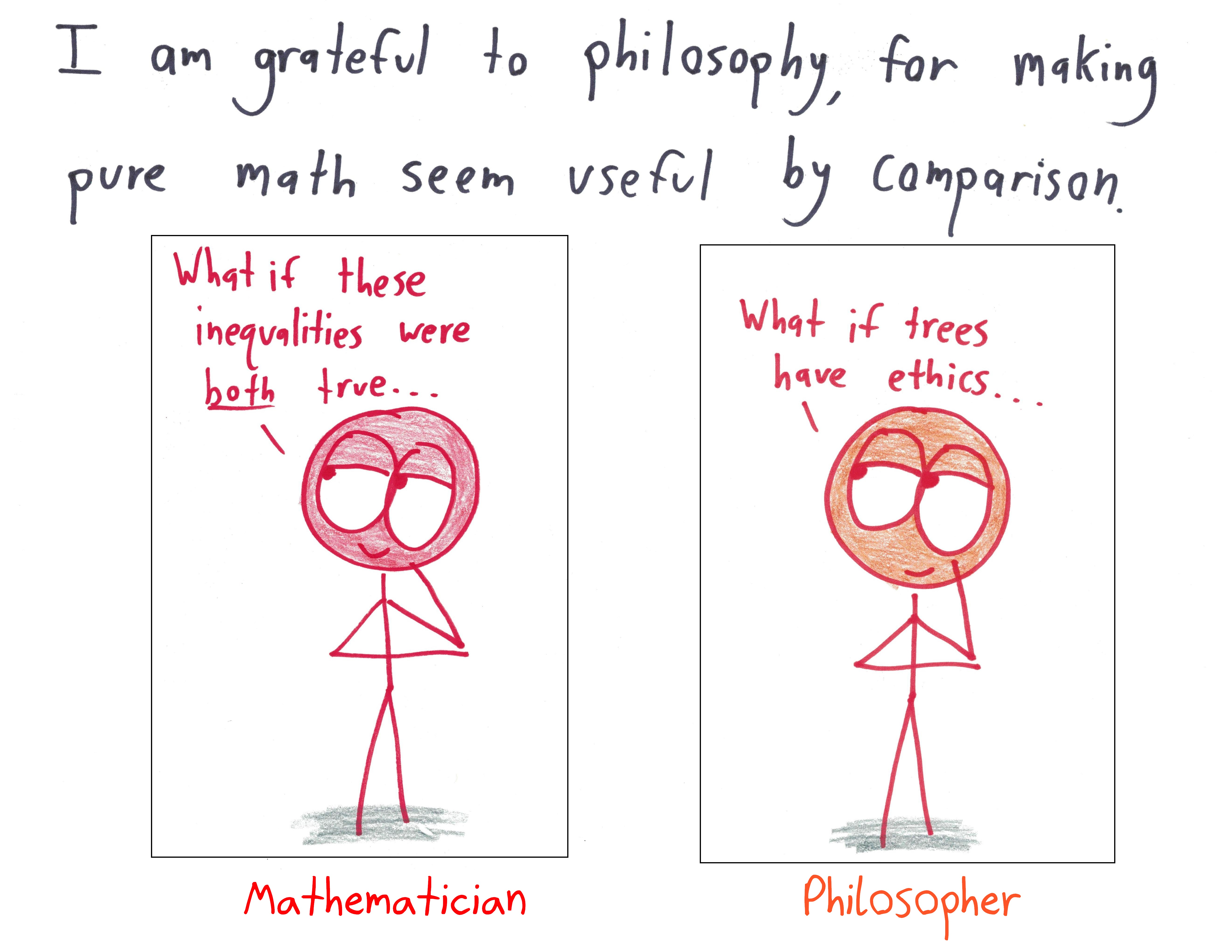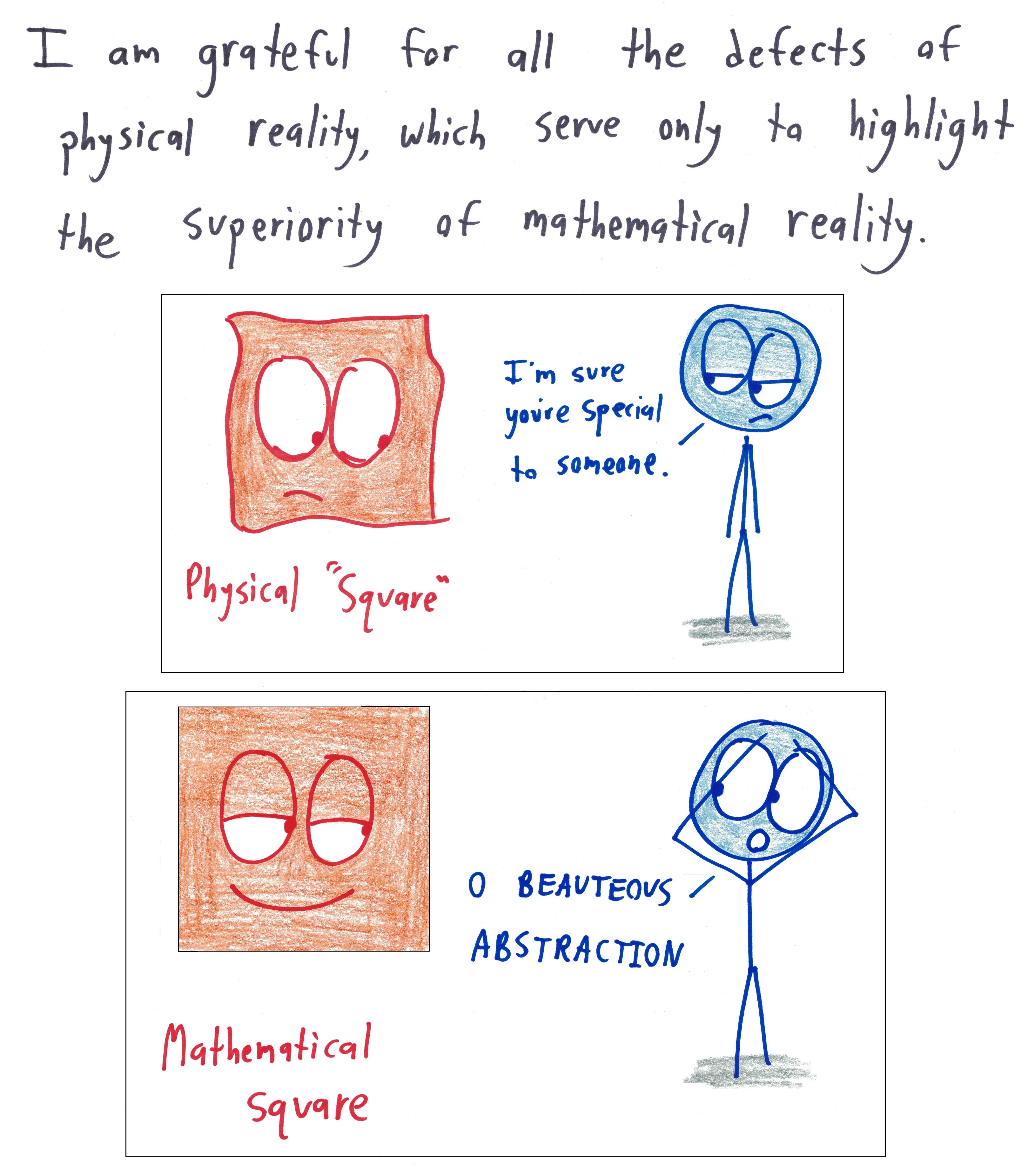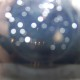# A Mathematician is Grateful For…## 16 thoughts on “A Mathematician is Grateful For…”

1.Kat says:

As a non-mathematician, (but I plan to go to re-take elementary school maths at the Khan Academy soon), I enjoyed these cartoons, and I can tell they’re clever, but didn’t understand more than one of them. Still hoping for someone to pick up the thread on my question of multiplying negative numbers. I feel once I understand that, I can trust you lot, and may eventually *get* some of these cartoons!

1.nhc says:

can you repost your question? don’t know where you originally posted it…

1.Ben Orlin says:

I just found it! It’s on the post “The Catchy Nonsense of ‘Two Negatives Make a Positive.'” I attempted an answer there, which I doubt will be satisfactory, in part because I find that curious people rarely find the answers to this question satisfactory!

2.Doug M says:

Let take a crack at multiplying negatives…

From the axioms of arithmattic.

a(b+1) = ab + a

(-a) is the additive inverse of a

(a + (-a)) = 0

And multiplication by 0 always gives 0.

a(0) = 0

So:
a(b + (-b)) = ab + (-ab) = a(0) = 0

-a(b + (-b)) = -ab + (-a)(-b) = 0

and (-a)(-b) must equal (ab)

And now for a completely different:

multiplication by (-1) completely flips the number-line. Everything that was on the right side of zero flips to the left side of zero, and everything on the left side of 0 flips to the right side of zero.

And now for a stupid one:

consider the function f(x) = kx with k not equal to 0.
This function is “injective” that is
if f(a) = f(b) then a=b
Injective functions are sometimes called 1-1 functions. For every output there is a unique input.
and if k is an integer, and a is an integer than f(a) must be an integer.

if k = -1,We map every positive integer to its corresponding negative integer. And every negative integer has a positive integer mapping to it. So where else are the negative numbers supposed to go! The function is still injective so \$f(a) = -a\$ must be mapping negative integers to positive integers.

3.Jennie Orr Thomas says:

Kat, Somebody down below tried to give you a bunch of algebraic proof of the “Multiplying two negatives gives a positive”. I bet that wasn’t what you were looking for. As a former theoretical mathematician that decided almost 50 years ago to go back to the “real world”, let’s try some real world examples instead. Double negatives in English mean a positive, as in “I didn’t not lie”. Think of negatives as the “opposite” of a positive and multiplication as repetitive addition. As in 3 x 3 = 3 + 3 + 3. If I add “-7” apples to a basket, that really means I took 7 apples out of the basket. If I did that 3 times, there would be 27 less apples in the basket or -7 x 3 = -21. Now think about what would happen if I “undid” removing 7 apples 3 times then I would have 21 MORE apples, -7 x -3 = 21. Does it make more sense to you now?

4.Jimmy says:

When you multiply negative numbers, the result is positive

2.Peter says:

Just sayin’, the common conception of what philosophers do is even more skewed than the conception of what mathematicians do.

3.xander says:

In reference to large numbers: I think I’ve encountered numbers as large as 5 a couple of times—I seem to recall some fiddly epsilon-over-five proof in an undergraduate course on functional analysis that I took once. But “7”? What the hell is a “7”?

4.mathtuition88 says:

Nice comics. Could anyone explain the “pull” door joke?

1.Greg says:

The joke is that pushing or pulling on the door actually has nothing to do with Euclidean or non-Euclidean space! The mathematician is just trying to cover for a dumb mistake (that we’ve all made too many times…) by using fancy terms to imply that it’s a mistake that an intelligent person would make.

1.mathtuition88 says:

Lol, that is really funny! I like the peer review process joke too. The slowness of peer review is a big insider joke for mathematicians.

5.mathtuition88 says:

Reblogged this on Singapore Maths Tuition.

6.gnnop says:

Great! (Your comics are always linked in the Iowa State University weekly math email)

7.paigeschronicles says:

these comics are so funny and cool!

8.Ingel Soong says: